# RBSE Maths Class 10 Chapter 11: Similarity Important Questions and Solutions

RBSE Class 10 Maths Chapter 11 – Similarity Important questions and solutions are provided here. All these questions given here have detailed explanations, along with suitable reasons. The RBSE Class 10 important questions and solutions available at BYJU’S has a full coverage of all the concepts.

Chapter 11 of RBSE Class 10 has four exercises which involve questions on similarity and congruence of triangles. Different theorems discussed in this chapter, such as Basic proportionality theorem, Pythagoras theorem, etc., which will help the students in their higher classes. Also, enough questions are given here for a better understanding of all the concepts.

### RBSE Maths Chapter 11: Exercise 11.1 Textbook Important Questions and Solutions

Question 1: State whether following statements are true or false.

(i) Two congruent figures are similar.

(ii) Two similar figures are congruent.

(lii) Two polygons having the same ratio of their corresponding sides are similar.

(iv) Two polygons are similar if their corresponding sides are proportional and corresponding angles are equal.

(v) Two polygons having corresponding angles equal are similar.

Solution:

(i) Two congruent figures are similar -True

(ii) Two similar figures are congruent – False

(iii) Two polygons having the same ratio of their corresponding sides are similar -False

(iv) Two polygons are similar if their corresponding sides are proportional and corresponding angles are equal – True

(v) Two polygons having corresponding angles equal are similar – False

### RBSE Maths Chapter 11: Exercise 11.2 Textbook Important Questions and Solutions

Question 2: The points D and E are on the sides AB and AC in a ΔABC, such that DE ∥ BC.

(i) If AD = 6 cm, DB = 9 cm and AE = 8 cm, then find the measure of AC.

(ii) If AD = 4x – 3, AE = 8x – 7, BD = 3x – 1 and CE = 5x – 3, then find the value of x.

Solution:

In the triangle ABC, DE ∥ BC.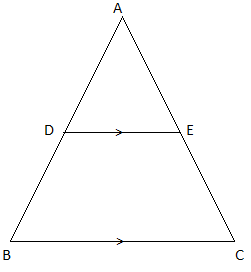(i) Given that, AD = 6 cm, DB = 9 cm and AE = 8 cm.

By Thales theorem (BPT),

6/9 = 8/(AC – AE)

6(AC – AE) = 8 × 9

6(AC – 8) = 72

AC – 8 = 72/6

AC = 12 + 8

AC = 20 cm

(ii) AD = 4x – 3, AE = 8x – 7, BD = 3x – 1 and CE = 5x – 3

By Thales theorem,

(4x – 3)/(3x – 1) = (8x – 7)/(5x – 3)

(4x – 3)(5x – 3) = (3x – 1)(8x – 7)

20x2 – 12x – 15x + 9 = 24x2 – 21x – 8x + 7

⇒ 24x2 – 20x2 – 29x + 27x + 7 – 9 = 0

⇒ 4x2 – 2x – 2 = 0

⇒ 2x2 – x – 1 = 0

⇒ 2x2 – 2x + x – 1 = 0

⇒ 2x(x – 1) + 1(x – 1) = 0

⇒ (2x + 1)(x – 1) = 0

⇒ 2x + 1 = 0, x – 1 = 0

⇒ x = -1/2, x = 1

Question 3: Points D and E are on the sides AB and AC respectively in the triangle ABC and the bases of the measures given below, state whether DE ∥ BC or not.

(i) AB = 12 cm, AD = 8 cm, AE = 12 cm and AC = 18 cm

(ii) AD = 10.5 cm, BD = 4.5 cm, AC = 4.8 cm and AE = 2.8 cm

Solution:

Given that, points D and E are on the sides AB and AC respectively in the triangle ABC.

(i) AB = 12 cm, AD = 8 cm, AE = 12 cm and AC = 18 cm

AE/CE = AE/(AC – AE)

AE/CE = 12/(18 – 12)

AE/CE = 12/6 = 2

/1

By the converse of BPT,

DE ∥ BC

(ii) AD = 10.5 cm, BD = 4.5 cm, AC = 4.8 cm and AE = 2.8 cm

AE/CE = AE/(AC – AE)

AE/CE = 2.8/(4.8 – 2.8)

AE/CE = 2.8/2 = 28/20

AE/CE = 7/5

Therefore, DE is not parallel to BC.

Question 4: In ΔABC, points D and E are respectively situated on AB and AC such that BD = CE. If ∠B = ∠C, then show that DE ∥ BC.

Solution:

Given,

In ∆ABC, D and E lie on sides AB and AC such that BD = CE and ∠B = ∠C.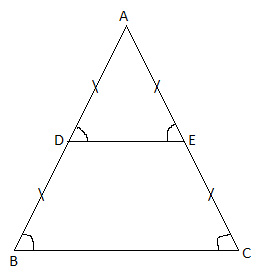In ∆ABC,

∠B = ∠C

AB = AC (opposite sides of equals angles are equal)

⇒ AD + DB = AE + CE (DB = CE)

∠D = ∠E (opposite angles of equal sides are equal)

Therefore, ∆ADE is an isosceles triangle.

Thus, ∆ABC and ∆ADE are isosceles triangles.

∠A = ∠A (common)

∴ ∠B = ∠C = ∠D = ∠E

∠B = ∠D (corresponding angles)

⇒ DE || BC

Question 5: In the figure, if DE ∥ BC and CD ∥ EF, then prove that AD2 = AB × AF.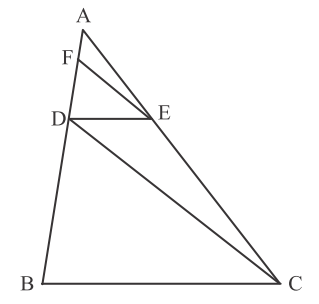Solution:

Given,

In triangle ABC, DE ∥ BC and CD ∥ EF.

By Basic proportionality theorem(BPT),

⇒ DB/AD = CE/AE (by inverse proportionality law)

⇒ (DB/AD) + 1 = (CE/AE) + 1

EF ∥ DC

By Basic proportionality theorem (BPT),

AF/FD = AE/CE

⇒ FD/AF = CE/AE

⇒ (FD/AF) + 1 = (CE/AE) + 1

⇒ (FD + AF)/AF = (CE + AE)/AE

From (i) and (ii),

⇒ AD2 = AF × AB

Question 6: In the figure, if EF ∥ DC ∥ AB, then prove that AE/ED = BF/FC.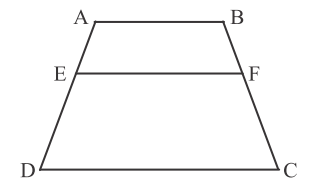Solution:

Given,

EF ∥ DC ∥ AB

Join AC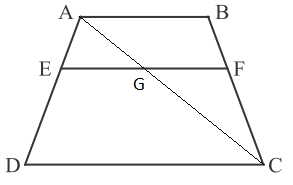By Basic proportionality theorem,

AE/ED = AG/GC ….(i)

In triangle ABC,

By Basic proportionality theorem,

CF/FB = CG/GA

or

BF/FC = AG/GC ….(ii)

From (i) and (ii),

AE/ED = BF/FC

Hence proved.

Question 7: ABCD is a parallelogram in which point P is situated on the side BC. If DP and AB are increased, then they meet at L. Prove that:

(i) DP/PL = DC/BL

(ii) DL/DP = AL/DC

Solution:

Given,

ABCD is a parallelogram in which point P is situated on the side BC.

Also, DP and AB are increased, then they meet at L.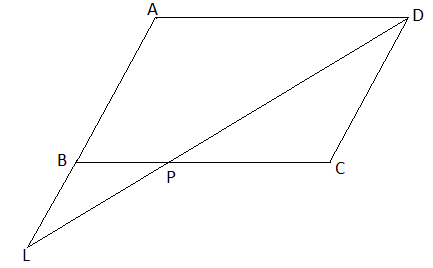By Basic proportionality theorem,

LP/DP = LB/AB ….(i)

or DP/LP = DC/BL (opposite sides of a parallelogram are equal)

⇒ DP/PL = DC/BL

Hence proved.

(ii) DL/DP = AL/DC

From (i),

LP/DP = LB/AB

(LP/DP) + 1 = (LB/AB) + 1

(LP + DP)/DP = (LB + AB)/AB

DL/LP = AL/AB

DL/DP = AL/CD (opposite sides of a parallelogram are equal)

Hence proved.

Question 8: Point D and E are situated in the side AB in a triangle ABC, such that AD = BE. If DP ∥ BC and EQ ∥ AC, then prove that PQ ∥ AB.

Solution:

Given,

Point D and E are situated in the side AB in a triangle ABC, such that AD = BE.

Also, DP ∥ BC and EQ ∥ AC.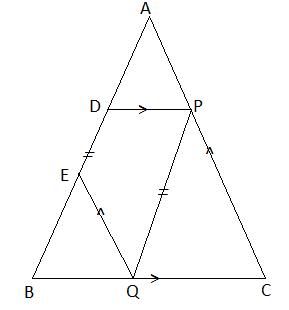In triangle ABC,

DP ∥ BC

By Basic proportionality theorem,

QE ∥ AC (given)

By Basic proportionality theorem,

BE/AE = BQ/QC ….(ii)

AD + DE = BE + DE

AE = BD ….(iv)

Substituting (iii) and (iv) in (ii),

AP/DB = AP/CP = BQ/QC

⇒ AP/CP = BQ/QC

⇒ CP/AP = QC/BQ

⇒ PQ ∥ AB

Hence proved.

Question 9: If the points D and E are situated on the sides AB and AC, respectively in a ΔABC such that BD = CE, then prove that ΔABC is an isosceles triangle.

Solution:

Given,

Points D and E are situated on the sides AB and AC respectively in a ΔABC such that BD = CE.

Join CD and BE.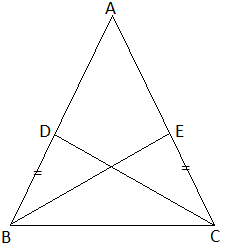In ΔBCD and ΔBEC,

BD = CE (given)

∠BDC = ∠BEC (angles opposite to equal sides)

BC = BC (common)

By SAS congruence rule,

ΔBCD ≅ ΔCBE

By CPCT,

∠ABC = ∠ACB

⇒ AB = AC (sides opposite to equal angles)

Therefore, ΔABC is an isosceles triangle.

Hence proved.

### RBSE Maths Chapter 11: Exercise 11.3 Textbook Important Questions and Solutions

Question 10: In ΔABC and ΔPQR, AB/PQ = BC/QR. State any two angles of two triangles which must be equal so that both triangles may be similar. Justify your answer.

Solution:

Given,

In ΔABC and ΔPQR, AB/PQ = BC/QR.

⇒ AB/BC = PQ/QR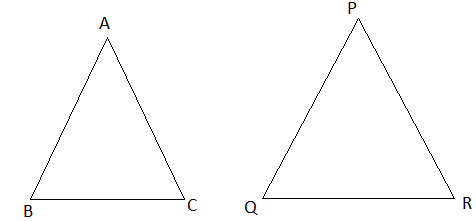∠B is the angle formed by sides AB and BC.

∠Q is the angle formed by sides PQ and QR.

∴ ∠B = ∠Q is required for similarity of two triangles.

Therefore, ∠B = ∠Q

AB/BC = PQ/QR

By SAS similarity rule,

∆ABC ~ ∆PQR

Question 11: In ∆ABC and ∆DEF, ∠A = ∠D, ∠B = ∠F, state whether ∆ABC ~ ∆DEF ? Give proper reason in support to your answer.

Solution:

No, the reason is, when ∠A = ∠D, ∠B = ∠F, then ∠C = ∠E.

Thus, ∆ABC ~ ∆DFE.

Question 12: If two sides of a triangle are proportional to two sides of another triangle also, one angle of the first triangle is equal to one angle of another triangle, then both triangles are similar. Is this statement true? Give reasons to support your answer.

Solution:

Given,

If two sides of a triangle are proportional to two sides of another triangle also, one angle of the first triangle is equal to one angle of another triangle, then both triangles are similar.

The given statement is not true because for similarity of two triangles, two corresponding sides and one included angle should be equal.

Question 13: What do you understand by equiangular triangles? What relation may exist among them?

Solution:

If the corresponding angles of two equiangular triangles are the same, then both triangles will be similar.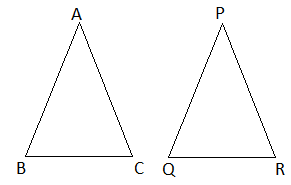In ∆ABC and ∆PQR

∠A = ∠P

∠B = ∠Q

and ∠C = ∠R

∴ ∆ABC ~ ∆PQR

Question 14: In the adjoining figure, ΔPRQ ~ ΔTRS, state which angles should be equal to each other in the given pair of similar triangles?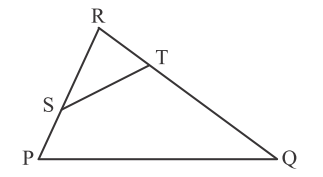Solution:

Given,

∆PRQ ~ ∆TRS

∴ ∠R = ∠R (common)

∠P = ∠RTS (corresponding angles)

∠Q = ∠RST (corresponding angles)

Therefore, the above pair of angles should be the same for the given pair of similar triangles.

Question 15: You have to choose two similar triangles out of the following triangles which are similar to each other if ∠CBE = ∠CAD.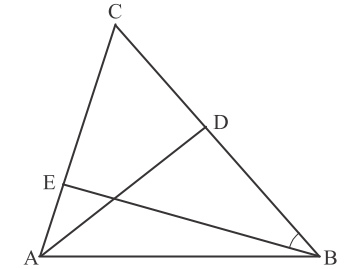Solution:

∠ACD = ∠BCE (common)

∴ ∆CAD ~ ∆CBE (by AA similarity rule)

Question 16: In the figure given below, PQ ∥ RS, then prove that ΔPOQ ~ ΔSOR.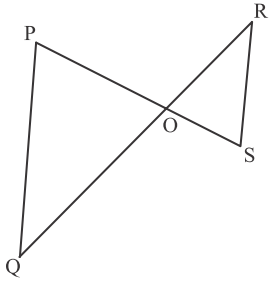Solution:

In ∆POQ and ∆SOR,

PQ || RS (given)

∠OPQ = ∠OSR (alternate angles)

∠POQ = ∠ROS (vertically opposite angles)

and ∠OQP = ∠ORS (alternate angles)

By AAA similarity criterion,

∆POQ ~ ∆SOR

Question 17: A girl of height 90 cm is walking away from the base of a long bulb’s pole at a speed of 1.2 m/s. If the bulb is put at the height of 3.6 m above the ground, find the length of her shadow after 4 minutes.

Solution:

Let AB be the length of the pole

CD be the height of the girl and DE be her shadow.

AB = 3.6 m

CD = 90 cm = 0.9 m

BD = Distance in 4 seconds

BD = 1.2 × 4 = 4.8 m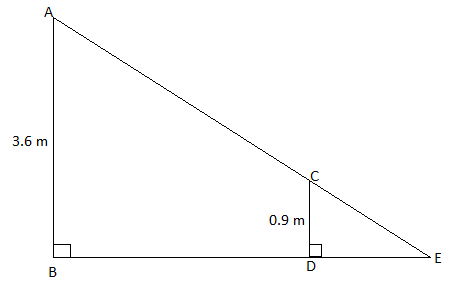In ∆ABE and ∆CDE,

∠B = ∠D (each 90°)

∠E = ∠E (comman)

∆ABE ~ ∆CDE (by AA criterion)

⇒ BE/DE = AB/CD

⇒ (4.8 + DE)/DE = 3.6/0.9

⇒ (4.8 + DE)/DE = 4

⇒ 4.8 + DE = 4DE

⇒ 4DE – DE = 4.8

⇒ 3DE = 4.8

⇒ DE = 4.8/3

⇒ DE = 1.6 m

Therefore, the length of the shadow of the girl after 4 seconds will be 1.6 m.

Question 18: The length of the shadow of a vertical pole 12 m high from the ground is 8 m. At the same time the shadow of the vertical minaret is 56 m long. Find the height of the minaret.

Solution:

Let AB be the minaret and CD be the vertical pole.

DE be the shadow of pole and BE be the shadow of minaret.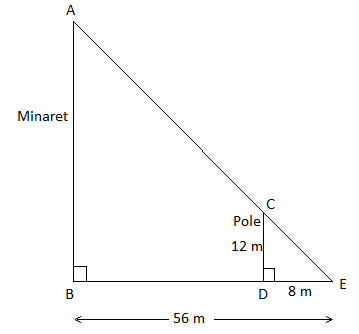In ∆ABE and ∆CDE,

∠B = ∠D (each 90°)

∠E = ∠E (comman)

∆ABE ~ ∆CDE (by AA criterion)

⇒ CD/AB = DE/BE

⇒ 12/AB = 8/56

⇒ AB = 12 × (56/8)

⇒ AB = 12 × 7

⇒ AB = 84 m

Therefore, the height of the tower is 84 m.

Question 19: If perpendicular is drawn from vertex A to opposite side BC in a triangle ABC, we get AD2 = BD × DC, then prove that ABC is a right angled triangle.

Solution:

Given,

Perpendicular from vertex A of ΔABC on side BC.

Also, AD2 = BD × DC

By SAS similarity criterion,

Therefore, ∠DAB = ∠DCA

∠ABD + ∠DCA + 90° = 180° (∠BAD = ∠DCA)

∠ABD + ∠BCA = 180° – 90° = 90°

In triangle ABC,

∠ABC + ∠BCA + ∠BAC = 180°

90° + ∠BAC = 180°

∠BAC = 180° – 90° = 90°

Therefore, triangle ABC is a right angled triangle.

Question 20: Prove that four triangles obtained by joining the mid-points of three sides of a triangle are similar to the original triangle.

Solution:

Let ABC be the triangle in which D, E, and F be the midpoints of sides AB, AC and BC, respectively.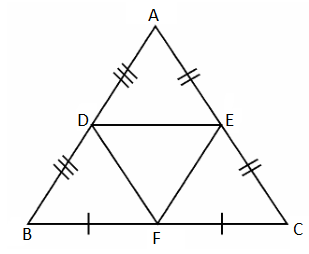Points D and E are midpoints of sides AB and AC.

∴ DE = (1/2) BC and DE || BC (by mid-point theorem) ….(i)

E and F are midpoints of AC and BC sides.

∴ EF = (1/2) AB and EF || AB ….(ii)

Point F and D are midpoints of sector BC and AB.

∴ FD = (1/2) AC and FD || AC ….(iii)

From (i) and (ii),

DE || BC and EF || AB

Therefore, DEFB is a parallelogram.

Diagonal DE divides the parallelogram DEFB into two congruent triangles.

∴ ∆DBF = ∆DEF ….(iv)

Diagonal EF divides the parallelogram DECF into two congruent triangles.

∴ ∆DEF = ∆DAE ….(v)

Also, diagonal EF divides the parallelogram DECF into two congruent triangles.

∴ ∆DEF = ∆EFC ….(iv)

From (iv), (v), and (vi),

∆DEF = ∆DAE = ∆EFC = ∆DBF (since DE || BC)

⇒ ∠C = ∠E

And ∠B = ∠D (corresponding angles)

Therefore, ∆ABC ~ ∆ADE ~ ∆BDF ~ ∆EFC

Hence proved.

Question 21: In the figure, if AB ⊥ BC, DC ⊥ BC and DE ⊥ AC, then prove that ΔCED ~ ΔABC.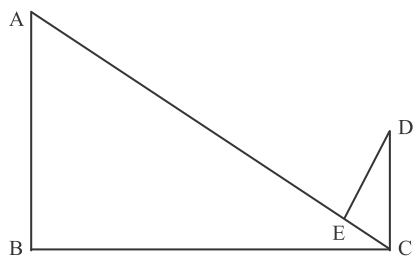Solution:

Given,

AB ⊥ BC, DC ⊥ BC and DE ⊥ AC

Consider,

AB ⊥ BC and DC ⊥ BC

∴ AB || DC

When AB || DC cuts by transversal AC

∠BAC = ∠ACD

or ∠BAC = ∠ECD ….(i)

∠B = ∠E = 90° (given) ….(ii)

From (i) and (ii) we can get ∠ACB = ∠EDC.

⇒ ∆ABC ~ ∆CED

Hence proved.

Question 22: In ΔABC, D is the midpoint of BC. If a line is drawn in such a way that it bisects AD and cut AD and AC at E and X respectively, then prove that: EX/BE = 1/3.

Solution:

Given,

D is the midpoint of BC in triangle ABC.

A line is drawn in such a way that it bisects AD and cut AD and AC at E and X respectively.

That means, AE = ED and BD = DC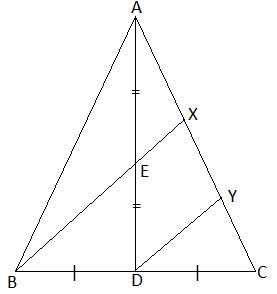Draw DY || BX meeting AC at Y.

In ∆BCX and ∆DCY,

∠BCX = ∠DCY (common)

∠BXC = ∠DYC (corresponding angles)

Thus, ∆BCX ~ ∆DCY (by AA similarity)

We know that the corresponding sides of similar triangles are proportional.

BX/DY = BC/DC = CX/CY

⇒ BX/DY = 2CD/CD (D is the midpoint of BC)

⇒ BX/DY = 2 ….(i)

Similarly,

⇒ EX/DY = AE/ED = 1/2 ….(ii) (E id the midpoint of AD)

Dividing (i) by (ii),

BX/EX = 2

⇒ BE + EX = 4EX

⇒ BE = 4EX – EX

⇒ BE = 3EX

⇒ EX/BE = 1/3

Hence proved.

### RBSE Maths Chapter 11: Exercise 11.4 Textbook Important Questions and Solutions

Question 23: State whether the following statements are true or false? Justify your answer if possible.

(i) If in ΔABC and ΔDEF, (area ΔABC)/(area ΔDEF) = AB2/DE2 = 9/4, then ΔABC ≅ ΔDEF.

(ii) Ratio of the areas of two similar triangles is equal to the ratio of the squares of their corresponding sides.

(iii) If ΔABC ~ ΔAXY and their areas are equal, then the sides XY and BC may coincide.

Solution:

(i) Given,

(area ΔABC)/(area ΔDEF) = AB2/DE2 = 9/4

⇒ AB/DE = 3/2

Ratio of the corresponding sides is 3 : 2

For congruency, the ratio should be 1 : 1.

Hence, the given statement is false.

(ii) Given,

Ratio of the areas of two similar triangles is equal to the ratio of the squares of their corresponding sides.

The above statement is true.

(iii) Given,

ΔABC ~ ΔAXY

Also,

(area ΔABC)/(area ΔAXY) = (AB)2/(AX)2 = (BC)2/(XY)2 = (AC)2/(AY)2

⇒ 1/1 = (AB)2/(AX)2 = (BC)2/(XY)2 = (AC)2/(AY)2

⇒ (AB)2/(AX)2 = 1/1

⇒ AB = AX

Similarly,

BC = XY

AC = AY

Thus, XY and BC will coincide.

Hence, the given statement is true.

Question 24: In ΔABC, DE ∥ BC and AD : DB = 2 : 3, find the ratio of the areas of ΔADE and ΔABC.

Solution:

Given,

In ΔABC, DE ∥ BC and AD : DB = 2 : 3.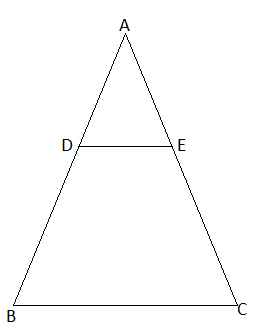∠B = ∠D

∠C = ∠E

By AA similarity criterion,

= (2/3)2 × [1/ (2/3) + 1]2

= (4/9) × [3/(2 + 3)]2

= (4/9) × (9/25)

= 4/25

Therefore, (area of ∆ADE)/(area of ∆ABC) = 4/25

Question 25: Perpendiculars PA and QB are drawn to two ends of a line segment AB. If P and Q are at the opposite sides of AB and their joining line intersects AB at O. Also, PO = 5 cm, QO = 7 cm, area of ΔPOB = 150 cm2, find the area of ΔQOA.

Solution:

Given information can be put in a diagram as shown below.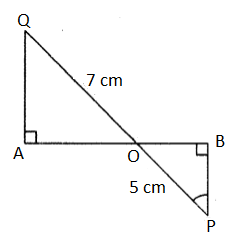Also, area of ΔPOB = 150 cm2

In ∆AOQ and ∆BOP,

∠A = ∠B = 90° (given)

∠AOQ = ∠POB (vertically opposite angles)

By AA similarity criterion,

∆AOQ ~ ∆BOP

(area of ΔAOQ)/(area of ΔBOP) = (OQ)2/(OP)2

(area of ΔAOQ)/150 = (7/5)2

(area of ΔAOQ) = (49/25) × 150

Area of ΔAOQ = 294 cm2

Question 26: In the figure, find the value of x in the terms of a, b and c.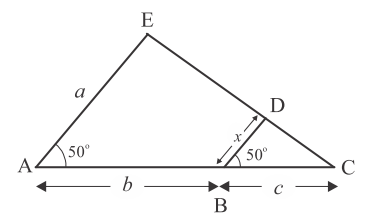Solution:

From the given figure,

∠A = ∠B = 50° (corresponding angles)

∴ AE || BD

In ∆BDC and ∆AEC,

∠A = ∠B = 50° (given)

∠E = ∠D (corresponding angles)

By AA similarity criterion,

∆BDC ~ ∆AEC

⇒ BC/AC = BD/AE

⇒ c/(b + c) = x/a

⇒ x = ac/(b + c)

Question 27: In ∆ABC, ∠B = 90° and BD ⊥ AC then prove that: ∆ADB ~ ∆BDC

Solution:

Given,

In ∆ABC, ∠B = 90° and BD ⊥ AC.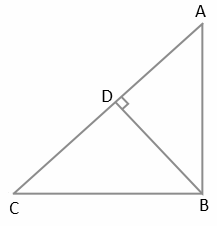In ∆ABC,

∠A + ∠C = 90° …..(i)

In ∆BDC,

∠DBC + ∠C = 90° [∵ ∠BDC = 90°] ….(ii)

From (i) and (ii),

∠A + ∠C = ∠DBC + ∠C

⇒ ∠A = ∠DBC ….(iii)

∠ADB = ∠BDC = 90° (given)

∠DAB = ∠DBC [From (iii)]

By AA similarity criterion,

Hence proved.

### RBSE Maths Chapter 11: Additional Important Questions and Solutions

Question 1: In the figure, DE ∥ AD, AD = 4 cm, DB = 6 cm, and AE = 5 cm, then measure of EC will be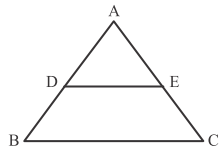(a) 6.5 cm

(b) 7.0 cm

(c) 7.5 cm

(d) 8.0 cm

Solution:

Given,

AE = 5 cm

BD = 6 cm

In ∆ABC,

BC || DE

By Basic proportionality theorem,

4/6 = 5/EC

⇒ EC = 5 × (6/4)

⇒ EC = 15/2

⇒ EC = 7.5 cm

Question 2: In the figure, AD is the bisector of ∠A, if AB = 6 cm, BD = 8 cm, DC = 6 cm, then the length of AC will be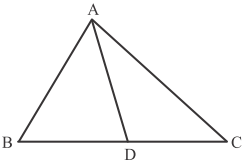(a) 4.0 cm

(b) 4.5 cm

(c) 5 cm

(d) 5.5 cm

Solution:

Given that, in ∆ABC, AD is the bisector of ∠A.

By Basic proportionality theorem,

AB/AC = BD/DC

6/AC = 8/6

⇒ AC = (6 × 6)/8

⇒ AC = 9/2

⇒ AC = 4.5 cm

Question 3: In the figure, if DE ∥ BC, then the value of x is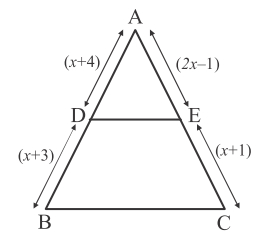(a) √5

(b) √6

(c) √3

(d) √7

Solution:

Given that, in ΔABC, DE || BC.

By Basic proportionality theorem,

(x + 4)/(x + 3) = (2x – 1)/(x + 1)

(x + 4)(x + 1) = (2x – 1)(x + 3)

⇒ x2 + 4x + x + 4 = 2x2 – x + 6x – 3

⇒ x2 + 5x + 4 = 2x2 + 5x – 3

⇒ x2 = 7

⇒ x = ± √7

Negative value of x is not possible for the given situation.

Therefore, x = √7

Question 4: In the figure, if AB = 3.4 cm, BD = 4 cm and BC = 10 cm, then the measure of AC is: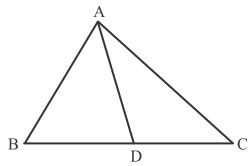(a) 5.1 cm

(b) 3.4 cm

(c) 6 cm

(d) 5.3 cm

Solution:

Given that, AD is the bisector of vertex angle A in triangle ABC.

⇒ AB/AC = BD/CD

⇒ AB/AC = BD/(BC – BD)

⇒ 3.4/AC = 4/(10 – 4)

⇒ 3.4/AC = 4/6

⇒ AC = (3.4 × 6)/4

⇒ AC = 1.7 × 3

⇒ AC = 5.1 cm

Question 5: The areas of two similar triangles are 25 cm2 and 36 cm2 respectively. If a median of smaller triangle is 10 cm, then the corresponding median of the larger triangle is:

(a) 12 cm

(b) 15 cm

(c) 10 cm

(d) 18 cm

Solution:

Let x cm be the measure of median of the larger triangle.

(area of smaller triangle)/(area of the larger triangle) = (median of smaller triangle)2/(median of the larger triangle)2

25/36 = (10)2/x2

25/36 = 100/x2

⇒ x2 = (100 36)/25

⇒ x2 = 144

⇒ x = √144

⇒ x = 12 cm

Question 6: In ∆ABC and ∆DEF ∠A = 50°, ∠B = 70°, ∠C = 60°, ∠D = 60°, ∠E = 70°, and ∠F = 50°. State which of the following is true?

(a) ∆ABC ~ ∆DEF

(b) ∆ABC ~ ∆EDF

(c) ∆ABC ~ ∆DFE

(d) ∆ABC ~ ∆FED

Solution:

From the given,

∠A = ∠F = 50°

∠B = ∠E = 70°

∠C = ∠D = 60°

∴ ∆ABC ~ ∆FED

Question 7: If ∆ABC ~ ∆DEF and AB = 10 cm, DE = 8 cm, then area of ∆ABC : area of ∆DEF will be:

(a) 25 : 16

(b) 16 : 25

(c) 4 : 5

(d) 5 : 4

Solution:

Given,

∆ABC ~ ∆DEF

(area of ∆ABC)/(area of ∆DEF) = (AB)2/(DE)2

= (10/8)2

= 100/64

= 25/16

Therefore, area of ∆ABC : area of ∆DEF = 25 : 16

Question 8: If ∆ABC ~ ∆DEF, also D and E points on the sides AB and AC such that DE || BC and AD = 8 cm, AB = 12 cm and AE = 12 cm, then the measure of CE is:

(a) 6 cm

(b) 18 cm

(c) 9 cm

(d) 15 cm

Solution:

Given that, DE || BC in triangle ABC.

By Basic proportionality theorem,

8/(12 – 8) = 12/CE

8/4 = 12/CE

2 = 12/CE

⇒ CE = 12/2

⇒ CE = 6 cm

Question 9: In a ΔABC, if point D is on the BC such that AB/AC = BD/DC and ∠B = 70°, ∠C = 50°, then find ∠BAD.

Solution:

In ∆ABC,

∠B = 70°, ∠C = 50°

By the angle sum property of a triangle,

∠A + ∠B + ∠C = 180°

∠A = 180° – (∠B + ∠C)

∠A = 180° – (70° + 50°)

∠A = 180° – 120°

∠A = 60°

AB/AC = BD/DC (given)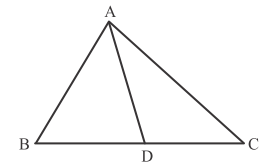Question 10: In ∆ABC, DE || BC also AD = 6 cm, DB = 9 cm and AE = 8 cm, find the length of AC.

Solution:

Given that, DE || BC in triangle ABC.

By Basic proportionality theorem,

6/9 = 8/CE

⇒ CE = (8 × 9)/6

⇒ CE = 12 cm

AC = AE + CE

= 8 + 12

= 20 cm

Therefore, the length of AC is 20 cm.

Question 11: If ΔABC ~ ΔDEF in which AB = 1.6 cm and DE = 2.4 cm, then find the ratio of the areas of ΔABC and ΔDEF.

Solution:

Given,

ΔABC ~ ΔDEF

(area of ΔABC)/(area of ΔDEF) = (AB)2/(DE)2

= (1.6/2.4)2

= (2/3)2

= 4/9

Therefore, area of ΔABC : area of ΔDEF = 4 : 9

Question 12: In the given figure, if OP.OQ = OR.OS, then show that ∠OPS = ∠ORQ and ∠OQR = ∠OSP.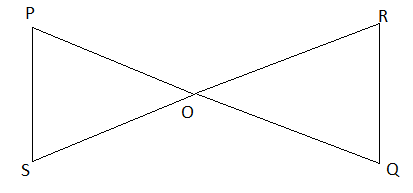Solution:

Given,

OP.OQ = OR.OS

⇒ OP/OR = OS/OQ

∠POS = ∠ROQ (vertically opposite angles)

Thus, ΔOPS ≅ ΔORQ (by SAS congruence rule)

We know that the corresponding angles of congruent triangles are equal.

∠OPS = ∠ORQ

∠OQR = ∠OSP

Question 13: If the ratio of corresponding medians of two similar triangles are 9 : 16, then the ratio of their areas.

Solution:

Given,

Ratio of medians of two similar triangles = 9 : 16

Ratio of area of two similar triangles = (Ratio of medians of two similar triangles)2

= (9/16)2

= 81/256

Ratio of area of two similar triangles = 81 : 256